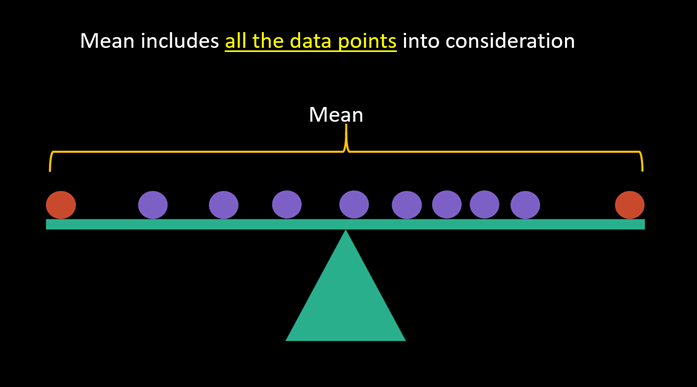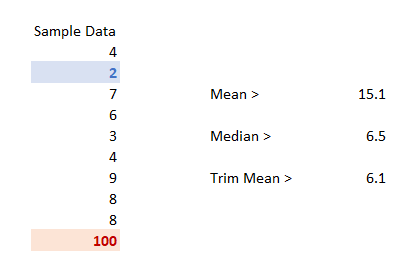top of page
Search

# Statistics Simplified# 6: Trim Mean

Statistics Simplified is the series to express statistics in layman terms.Photo by RF._.studio from Pexels

Got data points with special causes or outliers in your data set?

How to calculate the average in such cases?

A few common approaches are to either:

• exclude the record, or

• replace the extreme values with the median of the data set.

And there is another way: we use Trim Mean instead of a simple average.

## What is Trim Mean?

An average of the trimmed or “inner” data set.

### Which data points does it trim?

It excludes the data points from both ends.## TRIMMEAN in Excel

Excel has function TRIMMEAN which takes two arguments:

• Array: The data range

• Percent: The percentage of data points to be excluded from the calculation. It takes values from 0 to 1. If we say percent = 0.2, then it will exclude 20% of the dataset from the calculation. So, if the data set contains 10 data points, then it will exclude 2 data points; 1 from the top and 1 from the bottom (as demonstrated in the image above).

Just like the median, it first sorts the data into an order and then excludes the extreme data points from both ends.

In the example below, TRIMMEAN excluded 2 & 100 from the calculation, as I have supplied 0.2 as a percent.## Points to Remember

• TRIMMEAN function in Excel takes values greater than 0 and less than 1 in <percent>. Both 0 & 1 returns #NUM! error.

• TRIMMEAN excludes datapoints in the nearest multiples of 2. If the <percent> is .3 for the data set size of 10, it will remove 2 data points (one from the minimum and one from the maximum side).

Also, see:

Mean vs. Median

Geometric Mean

#vivran

bottom of page